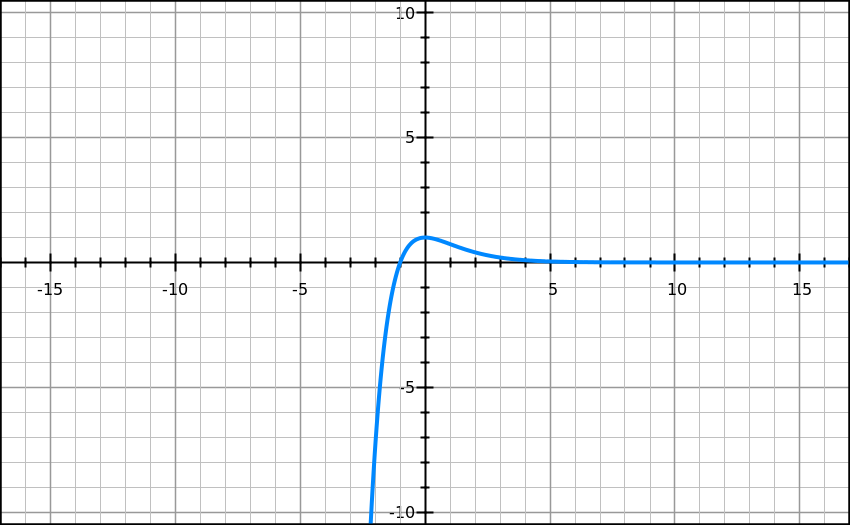# Damped oscillationsSuppose I have an oscillating system, that oscillates under a standard restoring force given by :

$F=-kx,$

where $x$ is the displacement and $k$ is the constant of proportionality with $k > 0$.

Now suppose it is being damped by a viscous force given by the standard equation of

$-b\dot { x } ={ F }_{ drag }.$

Suppose that the particle under the forces have mass $m$, and at $t = 0$ we have $x={ x }_{ 0 }$.
Then what is the limiting value of $b$ for which the particle will not cross the origin even once?

Details and Assumptions

• The graph of the particle will appear as shown.
• Only consider the positive side, x-axis is time and y-axis displacement
• m=1 kg
• K=1 N/m
• No driving force acts on the object.
×